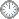# “执行定理”的证明 (方法c~f)

This is an in-mail from TYUST.
新入の者--> What is going on ? (redirected)new

(接前: 12 09 05) “执行定理” 的证明(方法).
.

---- 取 s 最大, 使 (X, B +sL) eps'-lc.

.

.

---- lct 的定义里有配对和线性系统.
---- 后两者是 "方" 和 "法" 的表现形式.
---- 故 lct 是由 “方法” 定义的数值.
---- 通过引入 s 和 eps', 将 lct 显化为 s 和 (X, B + sL) eps'-lc 的关系.
.

---- 预示新的操作.
.

(法嵌于方, 以方为主).

.

---- 给 eps' 赋予了结构, 也体现出新配对的特征.
.

.
* T 扮演什么角色？
---- 从形式上看, T 是由“方法” (X, B + sL) eps'-lc 定义.
---- 因此, T 部分地代表该方法.
(lct 和 s 的情况也类似于 T).
.

.
* 在 T 的中心上取一般点 x.
---- T 部分地代表 (X, B + sL) eps'-lc, 类似地, x 部分地代表 T.
.

.
* 按 x “非闭” 或 “闭” 两种情况讨论.
---- x 非闭的情况做两件事:
1) cut(A) 并运用归纳假设得: 存在正数 v, 使 (X, B + vL) lc 于 x 附近, 且 v 有正下界.
--- 由 A 打头, 体现出 “预办事, 法先行” 的道理.
--- 这里的主要输出是 (X, B + vL) lc.
--- 它是x附近的“方”(法).
2) (X, B) eps-lc 和 (X, B + vL) lc 做凸组合, 得到 (X, B + βvL) eps'-lc.
--- 由两个“方”的凸组合得到另一个“方”.
--- 新得的 (X, B + βvL) eps'-lc 跟开头的 (X, B + sL) eps'-lc 做系数比较, 得 s ≥ βv.
--- 不等式是“方”的一种形式.
.

.
---- x 闭的情况用到两个命题:
1）由命题 5.9, 得 (X, Λ) lc 于 x 附近.
--- Λ 非负 Q-divisor;
--- nΛ 是整的;
--- mA - Λ 系 ample;
--- T 是 (X, Λ) 的 lc place.
2）适当替换, 并假定 A - B - sL ample, 由命题 5.7 得
--- 存在自然数 q, 若ν: U --> X 是 resolution 使得 T 在 U 上, 则 μTν*L ≤ q.
.

--- 配对和不等式都是 “方” 的表现形式.
.

.
---- eps > eps'.

---- a(T, X, B) ≥ eps.

---- eps' = a(T, X, B + sL).

---- a(T, X, B) ≥ eps > eps' = a(T, X, B + sL).

.

---- 不等式往下走, 须得一法.
---- 再者, 尚需连接第五段末之方.
(这一条是个重要提示)
.
* 在 “主方” 两边做(减)“法”
---- a(T, X, B) - a(T, X, B + sL) ≥ eps - eps'.
==> μTν*sL ≥ eps - eps'.
==> s ≥ (eps - eps')/(μTν*L).
==> s ≥ (eps - eps')/q.
.

符号大全上下标.|| 常用：↑↓ π ΓΔΛΘΩμφΣ Ø∈  ∪ ∩ ⊆ ⊇ ⊂ ⊃ ≤ ≥ ⌊ ⌋ ⌈ ⌉ ≠ ≡ ⁻⁰ ¹ ² ³ ᵈ ₀ ₁ ₂ ₃ ᵢ .

#### Glossary(AG)

*

Introduction
....
....
11/9
...
Proposition 5.5 11/5

http://blog.sciencenet.cn/blog-315774-1202744.html

## 全部精选博文导读

GMT+8, 2019-11-19 06:21